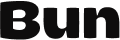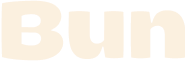# Mock functions in `bun test`

Create mocks with the `mock` function from `bun:test`.

``````import { test, expect, mock } from "bun:test";

const random = mock(() => Math.random());
``````

The mock function can accept arguments.

``````import { test, expect, mock } from "bun:test";

const random = mock((multiplier: number) => multiplier * Math.random());
``````

The result of `mock()` is a new function that's been decorated with some additional properties.

``````import { mock } from "bun:test";

const random = mock((multiplier: number) => multiplier * Math.random());

random(2);
random(10);

random.mock.calls;
// [[ 2 ], [ 10 ]]

random.mock.results;
//  [
//    { type: "return", value: 0.6533907460954099 },
//    { type: "return", value: 0.6452713933037312 }
//  ]
``````

These extra properties make it possible to write `expect` assertions about usage of the mock function, including how many times it was called, the arguments, and the return values.

``````import { test, expect, mock } from "bun:test";

const random = mock((multiplier: number) => multiplier * Math.random());

test("random", async () => {
const a = random(1);
const b = random(2);
const c = random(3);

expect(random).toHaveBeenCalled();
expect(random).toHaveBeenCalledTimes(3);
expect(random.mock.args).toEqual([, , ]);
expect(random.mock.results).toEqual({ type: "return", value: a });
});
``````

See Docs > Test Runner > Mocks for complete documentation on mocking with the Bun test runner.